Open in App
Not now

# Boutique Management System using Python-MySQL Connectivity

• Difficulty Level : Medium
• Last Updated : 19 Jan, 2023

In this article, we are going to make a simple project on a boutique management system using Python MySql connectivity.

## Introduction

This is a boutique management system made using MySQL connectivity with Python. It uses a MySQL database to store data in the form of tables and to maintain a proper record of all details. Different SQL queries that include DDL, DQL, DML, and DCL commands are used in this project which is useful to perform CRUD (Create, Read, Update, Delete) operations using MySQL. This management system provides a common platform for customers, employees, as well employers to use the services. The various functionalities provided in this system are:

Customers: Customer can create their accounts, sign in to their accounts and perform various operations such as book their orders, view booking, delete bookings and update their details.

Employees: Employees can sign in to their account using their employee id and perform operations such as updating delivered orders of customers, adding a new product, and deleting a product.

Employer: The employer can perform two operations which are viewing product details and adding a new employee.

## Prerequisites

MySQL should be installed on our system. Refer to this link to Download the MySQL installer. Once MySQL Server is installed properly, we can create databases and tables using MySQL commands in MySQL Command-Line-Client. MySQL Command Line Client is installed together with the MySQL Server, so you don’t need to download and install the MySQL client separately. To check whether you have the client program on your machine, go to search, and type “MySQL”. To access MySQL Server from the command-line client, open the program and enter the password after that, you will be able to use the client.

The tables used for this Boutique Management System are made using MySQL Command-Line-Client.

### Creating database

```CREATE DATABASE sboutique;
USE sboutique;```

### Creating customer table

The customer table has 6 columns, which store customer ID, customer name, last name, customer’s phone number, address of the customer, and products booked.

```create table customer (
cust_id int(8) PRIMARY KEY,
c_nam varchar(30),
c_lnam varchar(30),
c_phno varchar(10),
bkd_pro varchar(40) );```

Here cust_id is made the primary key because each customer is considered to be unique.

### Creating employee table

The employee table has 5 columns which stores, employee ID, employee name, last name, phone number, and address.

```create table employee (
emp_id char(3) PRIMARY KEY,
e_nam varchar(30),
e_lnam varchar(30),
e_phno varchar(10),

Here employee ID is made the primary key because each employee is considered to be unique.

### Creating products table

The products table has 4 columns with columns product number, product id, product price, and product stock.

```create table products (
pro_num char(5),
pro_id char(10) PRIMARY KEY,
pro_price int(6),
pro_stk int(5) );```

Here product ID is made as the primary key because each product in the database has a unique ID.

Let’s insert some products into the products table.

```INSERT INTO products VALUES
('1','KWPTP25',330,18),
('2','KWPTP30',450,30),
('3','KWPTP45',650,20),
('4','SSST025',850,10),
('5','SSST030',350,12);```

These 5 records are now added to the table.

Let’s check the description and contents in the table. Type the following command in MySQL Command-Line-Client.

## Checking the table structure and data stored in the table

```USE sboutique;
SHOW TABLES;```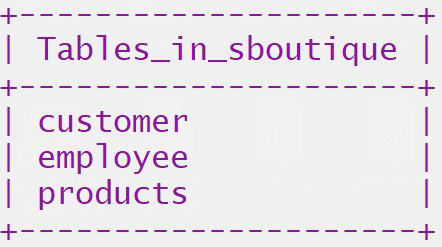Tables in sboutique database

`DESC customer;`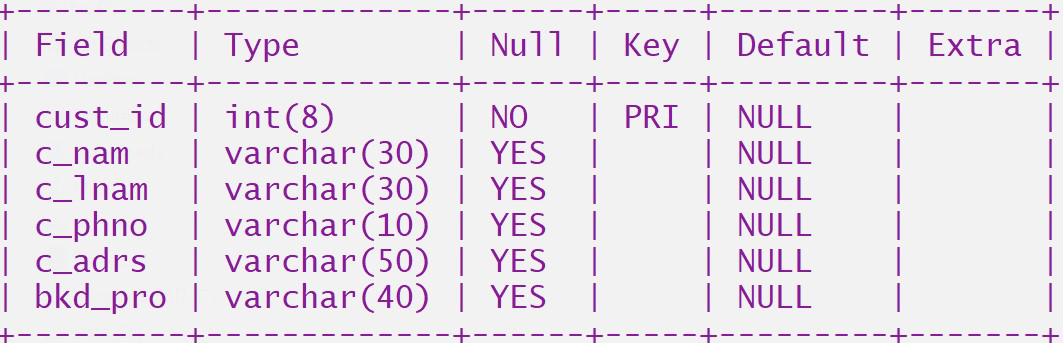Structure of customer table

`DESC employee;`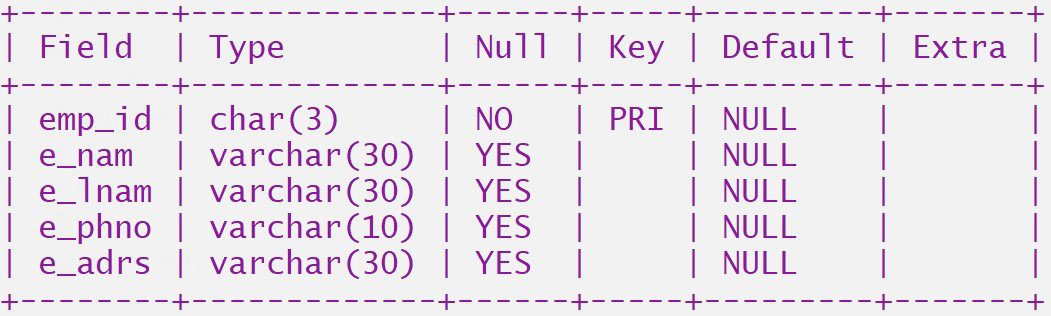Structure of employee table

`DESC products;`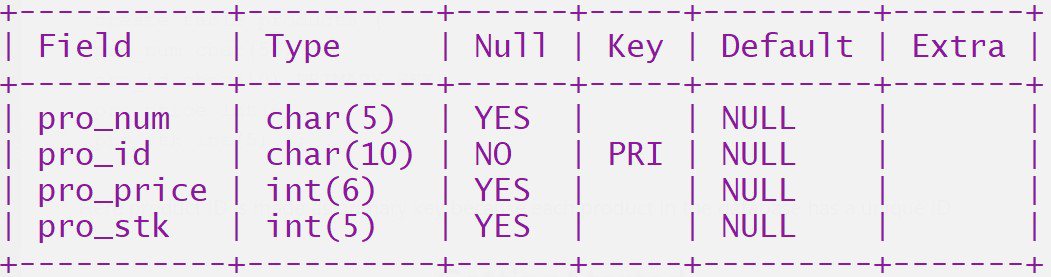Structure of products table

To view entered records in the table, execute the below command :

`SELECT * FROM products;`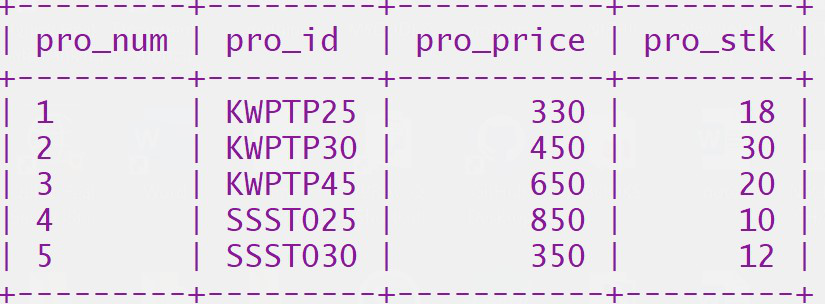Data in products table

Now the tables are created in the database. This database can be used to store create records, update values in tables, and delete records.

## Getting Started

We need to make a connection between the MySQL database and Python so that the tables can be accessed using python IDE. For making a connection we need to install mysql-connector which can be done by writing the following command in the command prompt on Windows.

`pip install mysql-connector-python`

After installation of the mysql-connector, we can connect MySQL with Python which can be done by writing the following code.

## Python3

 `# Importing mysql connector module``import` `mysql.connector``# Making MySQL connection object``mycon ``=` `mysql.connector.connect(``    ``host``=``'localhost'``, user``=``'root'``,``    ``password``=``'password'``,``    ``database``=``'sboutique'``)``# Making MySQL cursor object``mycur ``=` `mycon.cursor()`

In the above code, we have to enter our localhost address, port number, and password that we have set while installing MySQL workbench It will look like the below image after setup MySQL workbench also we have to enter the database name on which we worked on.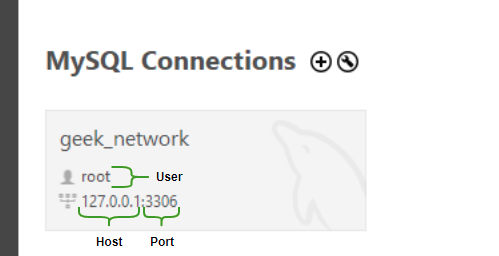## Database and Tables in Use :

The name of the MySQL Database used is “sboutique” and the following tables are used :## Functions in the program

### 1. Check() Function

This function is being used to return a list of IDs of all the customers in the boutique. At the time of a customer login or new customer, it is used to check if the customer with this ID already exists or not.

## Python3

 `# To check if a customer of a given ID exists or not``def` `check():``    ``# Query to select all customer IDs``    ``# from the table``    ``qry ``=` `'select cust_id from customer;'``    ` `    ``mycur.execute(qry)``    ``''' Return a list where each element``    ``in the list is a tuple``    ``fetched from each record in table``    ``Each tuple contains a single element``    ``as only customer IDs are fetched``    ``from cust_id column of each record'''``  ` `    ``d ``=` `mycur.fetchall()``    ``# To create a list of all customer IDs in the table``    ``list_of_ids ``=` `[]``    ``for` `ids ``in` `d:``        ``# A list of all customer IDs in table``        ``list_of_ids.append(ids[``0``])``        ` `    ``return` `list_of_ids`

### 2. Function to create a new customer account

The customer account function asks customers to enter their customer ID and it checks if a customer with this ID already exists or not because customer ID is a primary key in the customer table in the database and the values entered in these columns must be unique. If the customer exists it displays a message, else it takes the customer’s details and inserts the record in the customer table of the database.

## Python3

 `# To create a new account for the customer``def` `cust_ac():``    ``ask ``=` `'Y'``    ``list_of_ids ``=` `check()``    ``while` `ask ``in` `'yY'``:``        ``custid ``=` `int``(``input``(``'Enter your customer id...   '``))``        ``# to check if a customer already exists with this ID``        ``if` `custid ``in` `list_of_ids:``            ``print``('This Customer ``Id` `already exists....\``Try creating a new one')``        ``else``:``          ``# Tuple to contain details of the customer``            ``c_det ``=` `()``            ``cnam ``=` `input``(``'First Name : '``)``            ``clnam ``=` `input``(``'Last Name : '``)``            ``cphno ``=` `input``(``'Phone Number : '``)``            ``cadrs ``=` `input``(``'Your Address : '``)``            ``c_det ``=` `(custid, cnam, clnam, cphno, cadrs)``            ` `            ``''' Values inserted in the table and default NULL value are``                ``provided for booked product at the time of creation``                ``of customer account '''` `            ``qry ``=` `'insert into customer values(%s,%s,%s,%s,%s,NULL);'``            ` `            ``# value of the fields to be entered with the query``            ``val ``=` `c_det``            ` `            ``mycur.execute(qry, val)``            ``mycon.commit()``            ``print``(``'Customer details entered'``)``            ``ask ``=` `input``(``'Do you want to continue (Y/N) '``)``            ``if` `ask ``not` `in` `(``'Yy'``):``                ``space()``                ``break`

### 3. Get booked products function

This function returns a list of products booked by a customer using the customer’s ID. It is used in other functions to view or delete the booked orders of the customer.

## Python3

 `# To select all booked products of``# a given customer from the table``def` `get_bkd_pro(cust_id):``    ``qry ``=` `'select bkd_pro ``from` `customer\``where cust_id``=``%``s;'``    ``mycur.execute(qry, (cust_id,))``    ``bp ``=` `mycur.fetchone()``    ``bkd_pro ``=` `bp[``0``]``    ``return` `bkd_pro`

### 4. Function for Customer Login

This function allows the customer to log in to their accounts. It first checks if a customer with entered ID exists or not, and then asks for the customer’s choice to :

• View their booked products
• To view the products booked by customers, the get booked product function is used where customer ID is passed as an argument.
• It then checks if the customer has any bookings or not and then displays the result accordingly.
• If more than one product is booked, the product IDs are stored as a single value in the table separated by ‘_’. The fetched values are then split and printed.
•  Book a new product
• To book a new product, the function asks the customer to enter the product ID and then checks if the product with the given ID exists in the products table or not. It then adds the product to the booked products column of the customer’s table.
• If the customer already has a booked product, the new product ID is concatenated with the existing ID and again stored in the table.
• Update their existing details
• For the customer to update their account, the function displays the existing customer details and then asks them to enter the fields they want to update.
• Cancel booked products
• To cancel booked products the function asks for product ID and checks if it is booked or not and then deletes it accordingly.

## Python3

 `def` `sign_in():``    ``try``:``        ``ask ``=` `int``(``input``(``'Enter customer ID to sign in : '``))``        ``# Using check function to check whether this account exists or not``        ``list_of_ids ``=` `check()``        ``if` `ask ``in` `list_of_ids:``            ``while` `True``:``                ``print``(``''' Do you want to :                                                                ``                         ``1) View Bookings``                         ``2)  Book a product``                         ``3)  Update Self Details``                         ``4)  Cancel booked products``                            ``enter 'back' to exit '''``)``                 ``# Take choice of the customer``                ``ccc ``=` `input``(``'enter choice -  '``)``                ``if` `ccc ``=``=` `'1'``:``                    ``# Get booked product function is used where cutomer ID``                    ``# is passed as an argument` `                    ``s ``=` `get_bkd_pro(ask)``                    ``# To check if the column has any value``                    ``if` `s ``is` `None` `or` `s ``=``=` `' '``:``                        ``print``(``'you have not booked products yet'``)``                    ``else``:``                        ``''' If more than one products are booked,``                         ``their IDs are stored as a single value``                         ``separated by '_' so we have to split the``                         ``string to print each product ID.'''``                        ` `                        ``# d is a list containing product IDs``                        ``d ``=` `s.split(``'_'``)``                        ` `                        ``print``(``'Booked products'``)``                        ``for` `bkditems ``in` `d:``                            ``print``(bkditems)` `                ``if` `ccc ``=``=` `'2'``:` `                    ``# check if the product to be booked exists or not``                    ``qry ``=` `'select pro_id from products;'``                    ``mycur.execute(qry)``                    ``pro_list ``=` `mycur.fetchall()``                    ``''' contains a list where each element is a tuple fetched``                     ``from each record, the tuple contains values in the``                     ``column named pro_nam from products table.'''` `                     ``# empty list to store product IDs``                    ``list_of_products ``=` `[]``                    ``for` `i ``in` `pro_list:``                        ``list_of_products.append(i[``0``])` `                    ``# Take ID and quantity of product to be booked``                    ``pro_id ``=` `input``(``'Enter the product id to book products :  '``)``                    ``# To add booked product in the column,we first``                    ``# need to check if it already contains a value in it``                    ``if` `pro_id ``in` `list_of_products:``                        ``# Customer ID is given as value along with``                        ``# query to fetch booked product for the given ID``                        ``qry ``=` `'select bkd_pro from customer where cust_id=%s;'``                        ``mycur.execute(qry, (ask,))``                        ``pr ``=` `mycur.fetchone()``                        ``# prl is value fetched from table``                        ``prl ``=` `pr[``0``]   ``                        ``# When the column is empty the new product is to stored``                        ``if` `prl ``is` `None` `or` `prl ``=``=` `' '``:``                            ``qry ``=` `'update customer set bkd_pro=%s where cust_id=%s;'`                   `                            ``val ``=` `(pro_id``+``'_'``, ask)``                            ``mycur.execute(qry, val)``                            ``mycon.commit()``                            ``print``(``'Your Product is booked !!'``)``                            ` `                      ``''' If there already exists a value in bkd_pro column,``                        ``new value must be concatenated with the existing``                        ``one and again stored in the table'''``                      ` `                        ``else``:``                            ``prl1 ``=` `prl``+``pro_id``                            ``qry2 ``=` `'update customer set bkd_pro=%s where cust_id=%s;'``                            ``# val2 is the new value containing all booked products``                            ``# to be stored in the column``                            ``val2 ``=` `(prl1``+``'_'``, ask)``                            ``mycur.execute(qry2, val2)``                            ``mycon.commit()``                            ``print``(``'Your Product is booked !!'``)``                    ``else``:``                        ``print``('This product does ``not` `exists.\``Please write the correct product ``id``!')` `                ``if` `ccc ``=``=` `'3'``:` `                    ``qry ``=` `'select cust_id,c_nam,c_lnam,c_phno,c_adrs\``                        ``from` `customer where cust_id ``=``%``s'``                    ``mycur.execute(qry, (ask,))``                    ``# clist contains list of all values fetched``                    ``# in the form of a tuple for this customer ID``                    ``clist ``=` `mycur.fetchone()``                    ``# list of fields to be updated``                    ``flds ``=` `[``'Name'``, ``'Last Name'``, ``'Ph.No'``, ``'Address'``]``                    ``dic ``=` `{}``                    ``print``(``"Your existing record is :"``)``                    ``# The fetched details are stored in the form of key``                    ``# value pair in a dictionary``                    ``for` `i ``in` `range``(``4``):``                        ``dic[flds[i]] ``=` `clist[i``+``1``]``                        ``print``(i``+``1``, ``'    '``, flds[i], ``'  :  '``, clist[i``+``1``])` `                    ``for` `i ``in` `range``(``len``(clist)):``                        ``updtc ``=` `int``(``input``(``'enter choice to update '``))``                        ``upval ``=` `input``(``'enter'``+``flds[updtc``-``1``]``+``'   '``)``                    ``# Change the value corresponding to the required field``                        ``dic[flds[updtc``-``1``]] ``=` `upval``                        ``yn ``=` `input``(``                            ``'Do you want to update other details? y or n '``)``                        ``if` `yn ``in` `'Nn'``:``                            ``break``                    ``qry ``=` `'update customer ``set` `c_nam``=``%``s,c_lnam``=``%``s,c_phno``=``%``s,\``                    ``c_adrs``=``%``s where cust_id``=``%``s;'` `                    ``updtl ``=` `tuple``(dic.values())``+``(ask,)``                    ``# The value to be passed along with the query is a tuple``                    ``# containing updated details of the given customer ID``                    ``val ``=` `(updtl)``                    ``mycur.execute(qry, val)``                    ``mycon.commit()``                    ``print``(``'Your details are updated '``)` `                ``if` `ccc ``=``=` `'4'``:` `                    ``try``:``                        ``# To get the existing bookings``                        ``# Booked products in the table``                        ``bkd_pro ``=` `get_bkd_pro(ask)``                        ``print``(``'Your Booking(s)  : \n  '``, bkd_pro)``                        ``if` `bkd_pro ``is` `None` `or` `bkd_pro ``=``=` `' '``:``                            ``print``(``'you have no bookings to cancel'``)``                        ``else``:``                            ``cw ``=` `input``("To cancel ``all` `products; enter A \nOR \``enter the product code to cancel :  ")``                            ``if` `cw ``in` `'Aa'``:``                                ``qry ``=` `'update customer ``set` `bkd_pro``=``NULL\``                                ``where cust_id``=``%``s'` `                                ``mycur.execute(qry, (ask,))``                                ``mycon.commit()``                                ``print``(``'All bookings deleted'``)``                            ``elif` `cw ``in` `bkd_pro:``                                ``# If more than one products entered,``                                ``# split them on the basis of '_'``                                ``# x is a list containing all booked products``                                ``x ``=` `(bkd_pro[``0``:``-``1``]).split(``'_'``)``                                ` `                                ``# Delete the required product ID``                                ``x.remove(cw)``                                ``updt_pro ``=` `''``                                ``# Again concatenate each product ID``                                ``# in the list to store in the table``                                ``for` `item ``in` `x:``                                    ``updt_pro ``=` `updt_pro``+``item``+``'_'``                                ``qry ``=` `'update customer set bkd_pro=%s where cust_id=%s'``                                ``val ``=` `(updt_pro, ask)``                                ``mycur.execute(qry, val)``                                ``mycon.commit()``                                ``print``(``'Booking Cancelled !'``)``                    ``except` `Exception:``                        ``print``(``'Some problem in updating details.Try again'``)``                ``if` `ccc.lower() ``=``=` `'back'``:``                    ``print``(``"Successfully logged out"``)``                    ``space()``                    ``break``        ``else``:``            ``print``(``'This Account does not exist. '``)``    ``except` `Exception:``        ``print``(``'Some error occurred. Try Again'``)`

### 5. View Products function

This function fetches all existing products from the database and then displays them in the form of a table.

## Python3

 `# To fetch values from all columns of``# product table to get product details``def` `view_pro():``    ``qry ``=` `'select * from products;'``    ``mycur.execute(qry)``    ``d ``=` `mycur.fetchall()``    ``# contains list of all records``    ``dic ``=` `{}``    ``# Each record fetched is separated into a key value pair``    ``# and stored in the dictionary where product ID is the key``    ``for` `i ``in` `d:``        ``dic[i[``0``]] ``=` `i[``1``:]``    ``print``(``'_'``*``80``)``  ``# Printing the dictionary in the form of a table``    ``print``(``"{:<17} {:<22} {:<23} {:<19}"``.``format``(``        ``'Product id'``, ``'Product name'``, ``'Price'``, ``'Stock'``))``    ``print``(``'_'``*``80``)``    ``for` `k, v ``in` `dic.items():``        ``a, b, c ``=` `v``        ``print``(``"{:<17} {:<22} {:<23} {:<19}"``.``format``(k, a, b, c))``    ``print``(``'_'``*``80``)`

Add products function is used by the employees of the boutique to add new product details. It asks for a product number, product ID, price, and stock from the employee and enters a new record in the products table of the database.

## Python3

 `# To add a new product in Products table``def` `addpro():``    ``# Display list of products``    ``view_pro()  ``    ``n ``=` `int``(``input``(``'Enter no of items to insert  '``))``    ``for` `j ``in` `range``(n):``          ``# Initialize tuple to store``        ``# product details.``        ``t ``=` `()``        ``pronum ``=` `input``(``"Product No.  "``)``        ``proid ``=` `input``(``'Product ID :  '``)``        ``pprice ``=` `int``(``input``(``'Price : '``))``        ``pstk ``=` `int``(``input``(``'Stock : '``))``        ``t ``=` `(pronum, proid, pprice, pstk)``        ``# Using MySql query``        ``qry ``=` `'insert into products values(%s,%s,%s,%s);'``        ``val ``=` `t``        ``mycur.execute(qry, val)``        ``mycon.commit()``        ``print``(``"Product Added"``)`

### 7. Delete Product Function

This function is used by the employees of the boutique to delete product details. It asks for the product ID and then deletes the record from the products table of the database.

## Python3

 `# To delete a product from the table``def` `delpro():``    ``view_pro()``    ``delt ``=` `input``(``"Enter ID of product to be deleted"``)``    ``qry ``=` `'delete from products where pro_id=%s;'``    ``mycur.execute(qry, (delt,))``    ``mycon.commit()``    ``print``(``"Product is deleted"``)`

### 8. Function for employee login

This function is used for employees to login into their accounts. It allows employees to :

• Update the records of delivered products.
• Add a new product to the database
• Deletes a product from the database

## Python3

 `# For Employee Login``def` `emp_sign_in():``    ``try``:``        ``ask ``=` `input``(``'Enter id to sign in to the account :   '``)``        ``# To check if the employee with this ID exists or not.``        ``qry ``=` `'select emp_id from employee;'``        ``mycur.execute(qry)``        ``d ``=` `mycur.fetchall()``        ``lis ``=` `[]``        ``for` `i ``in` `d:``            ``lis.append(i[``0``])``        ``if` `ask ``not` `in` `lis:``            ``print``(``'Enter the correct id'``)``        ``else``:``            ``while` `True``:``                ``space()``                ``ccc ``=` `input``("``1.` `Update delivered records\n``                            ``2.` `Add a New Product \n``                            ``3.` `Delete a product \n``                            ``Enter  ``'Back'` `to logout:  ")``                ``if` `ccc ``=``=` `'1'``:``                    ``cust_id ``=` `input``(``'Enter customer id'``)``                    ``# Check if the customer has bookings or not``                    ``bkd_pro ``=` `get_bkd_pro(cust_id)``                    ``if` `bkd_pro ``is` `None` `or` `bkd_pro ``=``=` `' '``:``                        ``print``(``'This customer has no bookings '``)``                    ``else``:``                        ``print``(``'All booking(s):  '``, bkd_pro)``                        ``pro_id ``=` `input``('Enter product code to\``remove the delivered product   ')``                        ``# The product IDs are stored in the form of a``                        ``# single value separated by '_'.``                        ``if` `pro_id ``in` `bkd_pro:``                            ``x ``=` `(bkd_pro[``0``:``-``1``]).split(``'_'``)``                            ``# Returns a list of all booked products,``                            ``# then remove the delivered product from list``                            ``x.remove(pro_id)``                            ``# Concatenate the existing products using '_'``                            ``updt_pro ``=` `''``                            ``for` `i ``in` `x:``                                ``updt_pro ``=` `updt_pro``+``i``+``'_'``                            ``qry ``=` `'update customer ``set` `bkd_pro``=``%``s \``where cust_id``=``%``s;'``                            ``val ``=` `(updt_pro, cust_id)``                            ``mycur.execute(qry, val)``                            ``mycon.commit()``                            ``print``('Delivered product ``is` `removed\``from` `the database. ')``                        ``else``:``                            ``print``(``'enter the correct code'``)``                ``elif` `ccc ``=``=` `'2'``:``                    ``addpro()``                ``elif` `ccc ``=``=` `'3'``:``                    ``delpro()``                ``elif` `ccc.lower() ``=``=` `'back'``:``                    ``print``(``"Successfully logged out "``)``                    ``break``    ``except` `Exception:``        ``print``(``'Give the correct input'``)`

Add employee function allows the employer to add a new employee to the boutique and insert the records into the employee table of the database.

## Python3

 `# To add employee details``def` `addemp(): ``    ``qry ``=` `"select * from employee;"``    ``mycur.execute(qry)``    ``emp_list ``=` `mycur.fetchall()``    ``print``(``"List of Employees "``)``    ``for` `emp ``in` `emp_list:``        ``print``(``"Emp Id : "``, emp[``0``], ``"  Name :   "``, emp[``1``],``              ``"  Last Name : "``, emp[``2``], ``"  Phone No :  "``, emp[``3``])``    ``ne ``=` `[]``    ``n ``=` `int``(``input``(``'enter the no. of employees to add  '``))``    ``for` `i ``in` `range``(``1``, n``+``1``):``        ``t ``=` `()``        ``print``(``'enter employee id  '``)``        ``idd ``=` `int``(``input``(``str``(i)``+``')    '``))``        ``print``(``'Name  '``)``        ``nam ``=` `input``(``str``(i)``+``')    '``)``        ``print``(``'Last name  '``)``        ``lnam ``=` `input``(``str``(i)``+``')    '``)``        ``print``(``'Contact no.  '``)``        ``conno ``=` `int``(``input``(``str``(i)``+``')    '``))``        ``print``(``'Address  '``)``        ``adrs ``=` `input``(``str``(i)``+``')    '``)``        ``# A tuple containing details of an employee``        ``t ``=` `(idd, nam, lnam, conno, adrs)``        ``# List containing details of n number``        ``# of employees to be added``        ``ne ``=` `ne``+``[t, ]``    ``qry ``=` `'insert into employee values(%s,%s,%s,%s,%s);'``    ``# A list containing details of each employee``    ``# in the form of a tuple is to be passed along with the query``    ``for` `i ``in` `range``(``len``(ne)):``        ``val ``=` `ne[i]``        ``mycur.execute(qry, val)``        ``mycon.commit()``    ``print``(``'All Employee details added. '``)``    ``space()`

### 11. Function for employer login

This function is used for employer login and allows the employer to :

## Python3

 `# For employer login``def` `employer():``    ``while` `True``:``        ``print``()``        ``print``(``'''Enter Your Choice:                                                    ``                    ``1)View Product Details``                    ``2)Add  a New Employee``                      ``enter back to exit'''``)``        ``ccc ``=` `input``(``'Enter _____  '``)``        ``if` `ccc ``=``=` `'1'``:``            ``view_pro()``        ``if` `ccc ``=``=` `'2'``:``            ``addemp()``        ``if` `ccc.lower() ``=``=` `"back"``:``            ``break`

## Main Program

The program first asks for a choice of the user to enter as a customer, employee, or employer and calls the respective functions for the functioning of the program.

## Python3

 `print``(``'WELCOME !'``)``# Running a infinite loop``while` `True``:``    ``print``(``'''Are you a :                                                  ``   ``(A). Customer``   ``(B). Employee``   ``(C). Employer``   ``enter e to exit '''``)``    ``ch ``=` `input``(``'Enter -  '``)``    ``try``:``        ``if` `ch ``in` `'aA'``:``            ``print``(``" 1. Create Account\n 2.Sign In into existing account"``)``            ``choice ``=` `input``(``'enter-   '``)``            ``if` `choice ``=``=` `'1'``:``                ``cust_ac()``            ``elif` `choice ``=``=` `'2'``:``                ``sign_in()``            ``else``:``                ``print``(``'Enter correct choice'``)``        ``if` `ch ``in` `'bB'``:``            ``emp_sign_in()``        ``if` `ch ``in` `'cC'``:``            ``employer()``        ``elif` `ch.lower() ``=``=` `"e"``:``            ``print``(``"Thankyou for visiting !"``)``            ``break``    ``except` `Exception:``        ``print``(``'Give the right input'``)``    ``space()`

Complete Code:

## Python3

 `# Import MySql Connector``import` `mysql.connector``# making MySQL connection object``mycon ``=` `mysql.connector.connect(``  ``host``=``'localhost'``, user``=``'root'``,``  ``password``=``'password'``, database``=``'sboutique'``)``# making MySQL cursor object``mycur ``=` `mycon.cursor()` `# To provide blank spaces in the output``def` `space(): ``    ``for` `i ``in` `range``(``1``):``        ``print``()` `# To check if a customer of a given ID exists or not``def` `check():``    ``# query to select all customer IDs from the table``    ``qry ``=` `'select cust_id from customer;'``    ``mycur.execute(qry)``    ` `    ``''' a list where each element in the list is a tuple``    ``fetched from each record in table``    ``Each tuple contains a single element as only customer IDs are fetched``    ``from cust_id column of each record '''``    ` `    ``d ``=` `mycur.fetchall()``    ` `    ``# to create a list of all customer IDs in the table``    ``list_of_ids ``=` `[]``    ``for` `ids ``in` `d:``        ``# a list of all customer IDs in table``        ``list_of_ids.append(ids[``0``])``    ``return` `list_of_ids` `# To create a new account for the customer``def` `cust_ac():``    ``ask ``=` `'Y'``    ``list_of_ids ``=` `check()``    ``while` `ask ``in` `'yY'``:``        ``custid ``=` `int``(``input``(``'Enter your customer id...   '``))``        ``# to check if a customer already exists with this ID``        ``if` `custid ``in` `list_of_ids:``            ``print``('This Customer ``Id` `already exists....\``Try creating a new one')``        ``else``:``          ``# Tuple to contain details of the customer``            ``c_det ``=` `()``            ``cnam ``=` `input``(``'First Name : '``)``            ``clnam ``=` `input``(``'Last Name : '``)``            ``cphno ``=` `input``(``'Phone Number : '``)``            ``cadrs ``=` `input``(``'Your Address : '``)``            ``c_det ``=` `(custid, cnam, clnam, cphno, cadrs)``            ` `            ``''' Values inserted in the table and default NULL value are``                ``provided for booked product at the time of creation``                ``of customer account '''` `            ``qry ``=` `'insert into customer values(%s,%s,%s,%s,%s,NULL);'``            ` `            ``# value of the fields to be entered with the query``            ``val ``=` `c_det``            ` `            ``mycur.execute(qry, val)``            ``mycon.commit()``            ``print``(``'Customer details entered'``)``            ``ask ``=` `input``(``'Do you want to continue (Y/N) '``)``            ``if` `ask ``not` `in` `(``'Yy'``):``                ``space()``                ``break` `# To select all booked products of a given customer from the table``def` `get_bkd_pro(cust_id):``    ``qry ``=` `'select bkd_pro from customer where cust_id=%s;'``    ``mycur.execute(qry, (cust_id,))``    ``bp ``=` `mycur.fetchone()``    ``bkd_pro ``=` `bp[``0``]``    ``return` `bkd_pro`  `def` `sign_in():``    ``try``:``        ``ask ``=` `int``(``input``(``'Enter customer ID to sign in : '``))``        ``# Using check function to check whether this account exists or not``        ``list_of_ids ``=` `check()``        ``if` `ask ``in` `list_of_ids:``            ``while` `True``:``                ``print``(``''' Do you want to :                                                                ``                         ``1) View Bookings``                         ``2)  Book a product``                         ``3)  Update Self Details``                         ``4)  Cancel booked products``                            ``enter 'back' to exit '''``)``                 ``# Take choice of the customer``                ``ccc ``=` `input``(``'enter choice -  '``)``                ``if` `ccc ``=``=` `'1'``:``                    ``# Get booked product function is used where cutomer ID``                    ``# is passed as an argument` `                    ``s ``=` `get_bkd_pro(ask)``                    ``# To check if the column has any value``                    ``if` `s ``is` `None` `or` `s ``=``=` `' '``:``                        ``print``(``'you have not booked products yet'``)``                    ``else``:``                        ``''' If more than one products are booked,``                         ``their IDs are stored as a single value``                         ``separated by '_' so we have to split the``                         ``string to print each product ID.'''``                        ` `                        ``# d is a list containing product IDs``                        ``d ``=` `s.split(``'_'``)``                        ` `                        ``print``(``'Booked products'``)``                        ``for` `bkditems ``in` `d:``                            ``print``(bkditems)` `                ``if` `ccc ``=``=` `'2'``:` `                    ``# check if the product to be booked exists or not``                    ``qry ``=` `'select pro_id from products;'``                    ``mycur.execute(qry)``                    ``pro_list ``=` `mycur.fetchall()``                    ``''' contains a list where each element is a tuple fetched``                     ``from each record, the tuple contains values in the``                     ``column named pro_nam from products table.'''` `                     ``# empty list to store product IDs``                    ``list_of_products ``=` `[]``                    ``for` `i ``in` `pro_list:``                        ``list_of_products.append(i[``0``])` `                    ``# Take ID and quantity of product to be booked``                    ``pro_id ``=` `input``(``'Enter the product id to book products :  '``)``                    ``# To add booked product in the column,we first``                    ``# need to check if it already contains a value in it``                    ``if` `pro_id ``in` `list_of_products:``                        ``# Customer ID is given as value along with``                        ``# query to fetch booked product for the given ID``                        ``qry ``=` `'select bkd_pro from customer where cust_id=%s;'``                        ``mycur.execute(qry, (ask,))``                        ``pr ``=` `mycur.fetchone()``                        ``# prl is value fetched from table``                        ``prl ``=` `pr[``0``]   ``                        ``# When the column is empty the new product is to stored``                        ``if` `prl ``is` `None` `or` `prl ``=``=` `' '``:``                            ``qry ``=` `'update customer set bkd_pro=%s where cust_id=%s;'`                   `                            ``val ``=` `(pro_id``+``'_'``, ask)``                            ``mycur.execute(qry, val)``                            ``mycon.commit()``                            ``print``(``'Your Product is booked !!'``)``                            ` `                      ``''' If there already exists a value in bkd_pro column,``                        ``new value must be concatenated with the existing``                        ``one and again stored in the table'''``                      ` `                        ``else``:``                            ``prl1 ``=` `prl``+``pro_id``                            ``qry2 ``=` `'update customer set bkd_pro=%s where cust_id=%s;'``                            ``# val2 is the new value containing all booked products``                            ``# to be stored in the column``                            ``val2 ``=` `(prl1``+``'_'``, ask)``                            ``mycur.execute(qry2, val2)``                            ``mycon.commit()``                            ``print``(``'Your Product is booked !!'``)``                    ``else``:``                        ``print``('This product does ``not` `exists.\``Please write the correct product ``id``!')` `                ``if` `ccc ``=``=` `'3'``:` `                    ``qry ``=` `'select cust_id,c_nam,c_lnam,c_phno,c_adrs\``                        ``from` `customer where cust_id ``=``%``s'``                    ``mycur.execute(qry, (ask,))``                    ``# clist contains list of all values fetched``                    ``# in the form of a tuple for this customer ID``                    ``clist ``=` `mycur.fetchone()``                    ``# list of fields to be updated``                    ``flds ``=` `[``'Name'``, ``'Last Name'``, ``'Ph.No'``, ``'Address'``]``                    ``dic ``=` `{}``                    ``print``(``"Your existing record is :"``)``                    ``# The fetched details are stored in the form of key``                    ``# value pair in a dictionary``                    ``for` `i ``in` `range``(``4``):``                        ``dic[flds[i]] ``=` `clist[i``+``1``]``                        ``print``(i``+``1``, ``'    '``, flds[i], ``'  :  '``, clist[i``+``1``])` `                    ``for` `i ``in` `range``(``len``(clist)):``                        ``updtc ``=` `int``(``input``(``'enter choice to update '``))``                        ``upval ``=` `input``(``'enter'``+``flds[updtc``-``1``]``+``'   '``)``                    ``# Change the value corresponding to the required field``                        ``dic[flds[updtc``-``1``]] ``=` `upval``                        ``yn ``=` `input``(``                            ``'Do you want to update other details? y or n '``)``                        ``if` `yn ``in` `'Nn'``:``                            ``break``                    ``qry ``=` `'update customer ``set` `c_nam``=``%``s,c_lnam``=``%``s,c_phno``=``%``s,\``                    ``c_adrs``=``%``s where cust_id``=``%``s;'` `                    ``updtl ``=` `tuple``(dic.values())``+``(ask,)``                    ``# The value to be passed along with the query is a tuple``                    ``# containing updated details of the given customer ID``                    ``val ``=` `(updtl)``                    ``mycur.execute(qry, val)``                    ``mycon.commit()``                    ``print``(``'Your details are are updated '``)` `                ``if` `ccc ``=``=` `'4'``:` `                    ``try``:``                        ``# To get the existing bookings``                        ``# Booked products in the table``                        ``bkd_pro ``=` `get_bkd_pro(ask)``                        ``print``(``'Your Booking(s)  : \n  '``, bkd_pro)``                        ``if` `bkd_pro ``is` `None` `or` `bkd_pro ``=``=` `' '``:``                            ``print``(``'you have no bookings to cancel'``)``                        ``else``:``                            ``cw ``=` `input``("To cancel ``all` `products; enter A \nOR \``enter the product code to cancel :  ")``                            ``if` `cw ``in` `'Aa'``:``                                ``qry ``=` `'update customer ``set` `bkd_pro``=``NULL\``                                ``where cust_id``=``%``s'` `                                ``mycur.execute(qry, (ask,))``                                ``mycon.commit()``                                ``print``(``'All bookings deleted'``)``                            ``elif` `cw ``in` `bkd_pro:``                                ``# If more than one products entered,``                                ``# split them on the basis of '_'``                                ``# x is a list containing all booked products``                                ``x ``=` `(bkd_pro[``0``:``-``1``]).split(``'_'``)``                                ` `                                ``# Delete the required product ID``                                ``x.remove(cw)``                                ``updt_pro ``=` `''``                                ``# Again concatenate each product ID``                                ``# in the list to store in the table``                                ``for` `item ``in` `x:``                                    ``updt_pro ``=` `updt_pro``+``item``+``'_'``                                ``qry ``=` `'update customer set bkd_pro=%s where cust_id=%s'``                                ``val ``=` `(updt_pro, ask)``                                ``mycur.execute(qry, val)``                                ``mycon.commit()``                                ``print``(``'Booking Cancelled !'``)``                    ``except` `Exception:``                        ``print``(``'Some problem in updating details.Try again'``)``                ``if` `ccc.lower() ``=``=` `'back'``:``                    ``print``(``"Successfully logged out"``)``                    ``space()``                    ``break``        ``else``:``            ``print``(``'This Account does not exist. '``)``    ``except` `Exception:``        ``print``(``'Some error occurred. Try Again'``)`  `# To fetch values from all columns of``# product table to get product details``def` `view_pro():``    ``qry ``=` `'select * from products;'``    ``mycur.execute(qry)``    ``d ``=` `mycur.fetchall()``    ``# contains list of all records``    ``dic ``=` `{}``    ``# Each record fetched is separated into a key value pair``    ``# and stored in the dictionary where product ID is the key``    ``for` `i ``in` `d:``        ``dic[i[``0``]] ``=` `i[``1``:]``    ``print``(``'_'``*``80``)``  ``# Printing the dictionary in the form of a table``    ``print``(``"{:<17} {:<22} {:<23} {:<19}"``.``format``(``        ``'Product id'``, ``'Product name'``, ``'Price'``, ``'Stock'``))``    ``print``(``'_'``*``80``)``    ``for` `k, v ``in` `dic.items():``        ``a, b, c ``=` `v``        ``print``(``"{:<17} {:<22} {:<23} {:<19}"``.``format``(k, a, b, c))``    ``print``(``'_'``*``80``)`  `# To add a new product in Products table``def` `addpro():``    ``# Display list of products``    ``view_pro()  ``    ``n ``=` `int``(``input``(``'Enter no of items to insert  '``))``    ``for` `j ``in` `range``(n):``          ``# Initialize tuple to store``        ``# product details.``        ``t ``=` `()``        ``pronum ``=` `input``(``"Product No.  "``)``        ``proid ``=` `input``(``'Product ID :  '``)``        ``pprice ``=` `int``(``input``(``'Price : '``))``        ``pstk ``=` `int``(``input``(``'Stock : '``))``        ``t ``=` `(pronum, proid, pprice, pstk)``        ``# Using MySql query``        ``qry ``=` `'insert into products values(%s,%s,%s,%s);'``        ``val ``=` `t``        ``mycur.execute(qry, val)``        ``mycon.commit()``        ``print``(``"Product Added"``)`  `# To delete a product from the table``def` `delpro():``    ``view_pro()``    ``delt ``=` `input``(``"Enter ID of product to be deleted"``)``    ``qry ``=` `'delete from products where pro_id=%s;'``    ``mycur.execute(qry, (delt,))``    ``mycon.commit()``    ``print``(``"Product is deleted"``)` `# For Employee Login``def` `emp_sign_in():``    ``try``:``        ``ask ``=` `input``(``'Enter id to sign in to the account :   '``)``        ``# To check if the employee with this ID exists or not.``        ``qry ``=` `'select emp_id from employee;'``        ``mycur.execute(qry)``        ``d ``=` `mycur.fetchall()``        ``lis ``=` `[]``        ``for` `i ``in` `d:``            ``lis.append(i[``0``])``        ``if` `ask ``not` `in` `lis:``            ``print``(``'Enter the correct id'``)``        ``else``:``            ``while` `True``:``                ``space()``                ``ccc ``=` `input``("``1.` `Update delivered records\n``                            ``2.` `Add a New Product \n``                            ``3.` `Delete a product \n``                            ``Enter  ``'Back'` `to logout:  ")``                ``if` `ccc ``=``=` `'1'``:``                    ``cust_id ``=` `input``(``'Enter customer id'``)``                    ``# Check if the customer has bookings or not``                    ``bkd_pro ``=` `get_bkd_pro(cust_id)``                    ``if` `bkd_pro ``is` `None` `or` `bkd_pro ``=``=` `' '``:``                        ``print``(``'This customer has no bookings '``)``                    ``else``:``                        ``print``(``'All booking(s):  '``, bkd_pro)``                        ``pro_id ``=` `input``('Enter product code to\``remove the delivered product   ')``                        ``# The product IDs are stored in the form of a``                        ``# single value separated by '_'.``                        ``if` `pro_id ``in` `bkd_pro:``                            ``x ``=` `(bkd_pro[``0``:``-``1``]).split(``'_'``)``                            ``# Returns a list of all booked products,``                            ``# then remove the delivered product from list``                            ``x.remove(pro_id)``                            ``# Concatenate the existing products using '_'``                            ``updt_pro ``=` `''``                            ``for` `i ``in` `x:``                                ``updt_pro ``=` `updt_pro``+``i``+``'_'``                            ``qry ``=` `'update customer ``set` `bkd_pro``=``%``s \``where cust_id``=``%``s;'``                            ``val ``=` `(updt_pro, cust_id)``                            ``mycur.execute(qry, val)``                            ``mycon.commit()``                            ``print``('Delivered product ``is` `removed\``from` `the database. ')``                        ``else``:``                            ``print``(``'enter the correct code'``)``                ``elif` `ccc ``=``=` `'2'``:``                    ``addpro()``                ``elif` `ccc ``=``=` `'3'``:``                    ``delpro()``                ``elif` `ccc.lower() ``=``=` `'back'``:``                    ``print``(``"Successfully logged out "``)``                    ``break``    ``except` `Exception:``        ``print``(``'Give the correct input'``)` `# To add employee details``def` `addemp(): ``    ``qry ``=` `"select * from employee;"``    ``mycur.execute(qry)``    ``emp_list ``=` `mycur.fetchall()``    ``print``(``"List of Employees "``)``    ``for` `emp ``in` `emp_list:``        ``print``(``"Emp Id : "``, emp[``0``], ``"  Name :   "``, emp[``1``],``              ``"  Last Name : "``, emp[``2``], ``"  Phone No :  "``, emp[``3``])``    ``ne ``=` `[]``    ``n ``=` `int``(``input``(``'enter the no. of employees to add  '``))``    ``for` `i ``in` `range``(``1``, n``+``1``):``        ``t ``=` `()``        ``print``(``'enter employee id  '``)``        ``idd ``=` `int``(``input``(``str``(i)``+``')    '``))``        ``print``(``'Name  '``)``        ``nam ``=` `input``(``str``(i)``+``')    '``)``        ``print``(``'Last name  '``)``        ``lnam ``=` `input``(``str``(i)``+``')    '``)``        ``print``(``'Contact no.  '``)``        ``conno ``=` `int``(``input``(``str``(i)``+``')    '``))``        ``print``(``'Address  '``)``        ``adrs ``=` `input``(``str``(i)``+``')    '``)``        ``# A tuple containing details of an employee``        ``t ``=` `(idd, nam, lnam, conno, adrs)``        ``# List containing details of n number``        ``# of employees to be added``        ``ne ``=` `ne``+``[t, ]``    ``qry ``=` `'insert into employee values(%s,%s,%s,%s,%s);'``    ``# A list containing details of each employee``    ``# in the form of a tuple is to be passed along with the query``    ``for` `i ``in` `range``(``len``(ne)):``        ``val ``=` `ne[i]``        ``mycur.execute(qry, val)``        ``mycon.commit()``    ``print``(``'All Employee details added. '``)``    ``space()`  `# For employer login``def` `employer(): ``    ``while` `True``:``        ``print``()``        ``print``(``'''Enter Your Choice:                                                    ``                    ``1)View Product Details``                    ``2)Add  a New Employee``                      ``enter back to exit'''``)``        ``ccc ``=` `input``(``'Enter _____  '``)``        ``if` `ccc ``=``=` `'1'``:``            ``view_pro()``        ``if` `ccc ``=``=` `'2'``:``            ``addemp()``        ``if` `ccc.lower() ``=``=` `"back"``:``            ``break`  `print``(``'WELCOME !'``)``# Running a infinite loop``while` `True``:``    ``print``(``'''Are you a :                                                  ``   ``(A). Customer``   ``(B). Employee``   ``(C). Employer``   ``enter e to exit '''``)``    ``ch ``=` `input``(``'Enter -  '``)``    ``try``:``        ``if` `ch ``in` `'aA'``:``            ``print``(``" 1. Create Account\n 2.Sign In into existing account"``)``            ``choice ``=` `input``(``'enter-   '``)``            ``if` `choice ``=``=` `'1'``:``                ``cust_ac()``            ``elif` `choice ``=``=` `'2'``:``                ``sign_in()``            ``else``:``                ``print``(``'Enter correct choice'``)``        ``if` `ch ``in` `'bB'``:``            ``emp_sign_in()``        ``if` `ch ``in` `'cC'``:``            ``employer()``        ``elif` `ch.lower() ``=``=` `"e"``:``            ``print``(``"Thankyou for visiting !"``)``            ``break``    ``except` `Exception:``        ``print``(``'Give the right input'``)``    ``space()`

Output:

Let’s view the records added to the database.

## Records in the Tables after executing the program:

Customer table: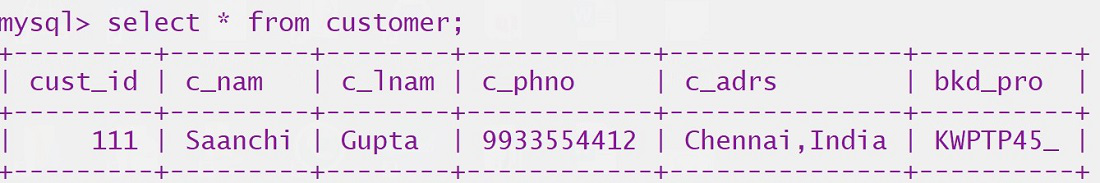Employee table: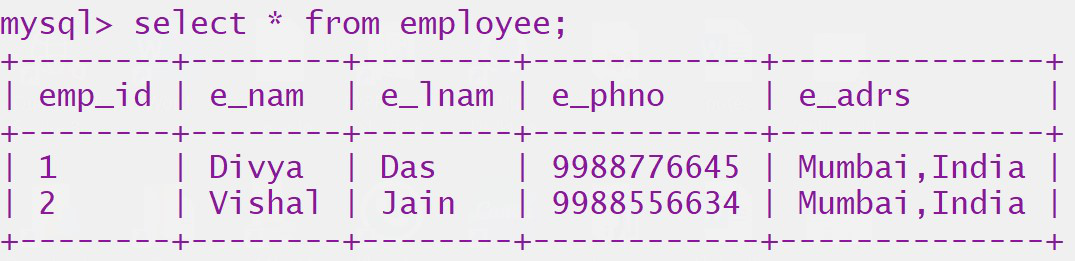Product table: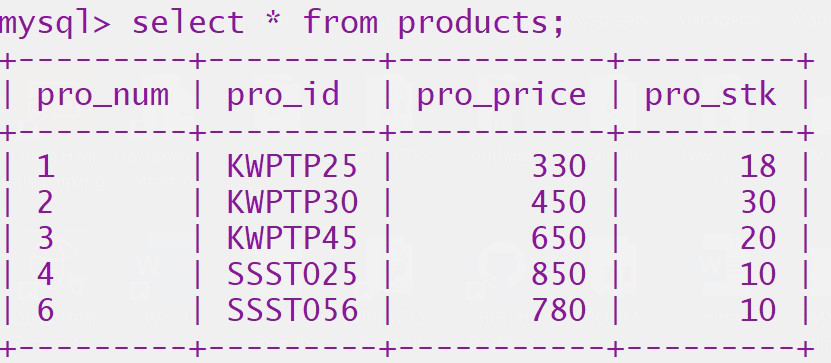My Personal Notes arrow_drop_up